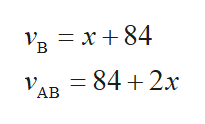Two planes are 1470 mi apart and traveling toward each other. One plane is traveling 80 mph faster than the other plane. The planes pass each other in 1.5 h. Find the speed of each plane.slower plane    mphfaster planemph A solution that is 20% salt is mixed with a solution that is 30% salt. Which of the following are not possible choices for the concentration of salt in the resulting mixture? (Select all that apply.)20%25%27%30%35% Rubbing alcohol is typically diluted with water to 70% strength. If you need 2.8 oz of 45% rubbing alcohol, how many ounces of 70% rubbing alcohol and how much water should you combine? alcohol     ozwater     oz Let q {-9, 0, 8}. Evaluate |q| for each element of the set.____(first element)_____(second element)_____(third element)

Question

Two planes are 1470 mi apart and traveling toward each other. One plane is traveling 80 mph faster than the other plane. The planes pass each other in 1.5 h. Find the speed of each plane.

 slower plane mph faster plane mph

A solution that is 20% salt is mixed with a solution that is 30% salt. Which of the following are not possible choices for the concentration of salt in the resulting mixture? (Select all that apply.)

20%
25%
27%
30%
35%

Rubbing alcohol is typically diluted with water to 70% strength. If you need 2.8 oz of 45% rubbing alcohol, how many ounces of 70% rubbing alcohol and how much water should you combine?
 alcohol oz water oz

Let q {-9, 0, 8}. Evaluate |q| for each element of the set.
____(first element)
_____(second element)
_____(third element)

Step 1

There are two planes named as A and B which are 1470 miles apart therefore,

And time taken in passing each is 1.5 hr so,

Step 2

Let the speed of one of the plane is denoted as follows:

Step 3

Since speed of other plane is 8...help_outlineImage Transcriptionclose— х +84 В - 84 + 2х АВ fullscreen

Want to see the full answer?

See Solution

Want to see this answer and more?

Our solutions are written by experts, many with advanced degrees, and available 24/7

See Solution
Tagged in

Algebra